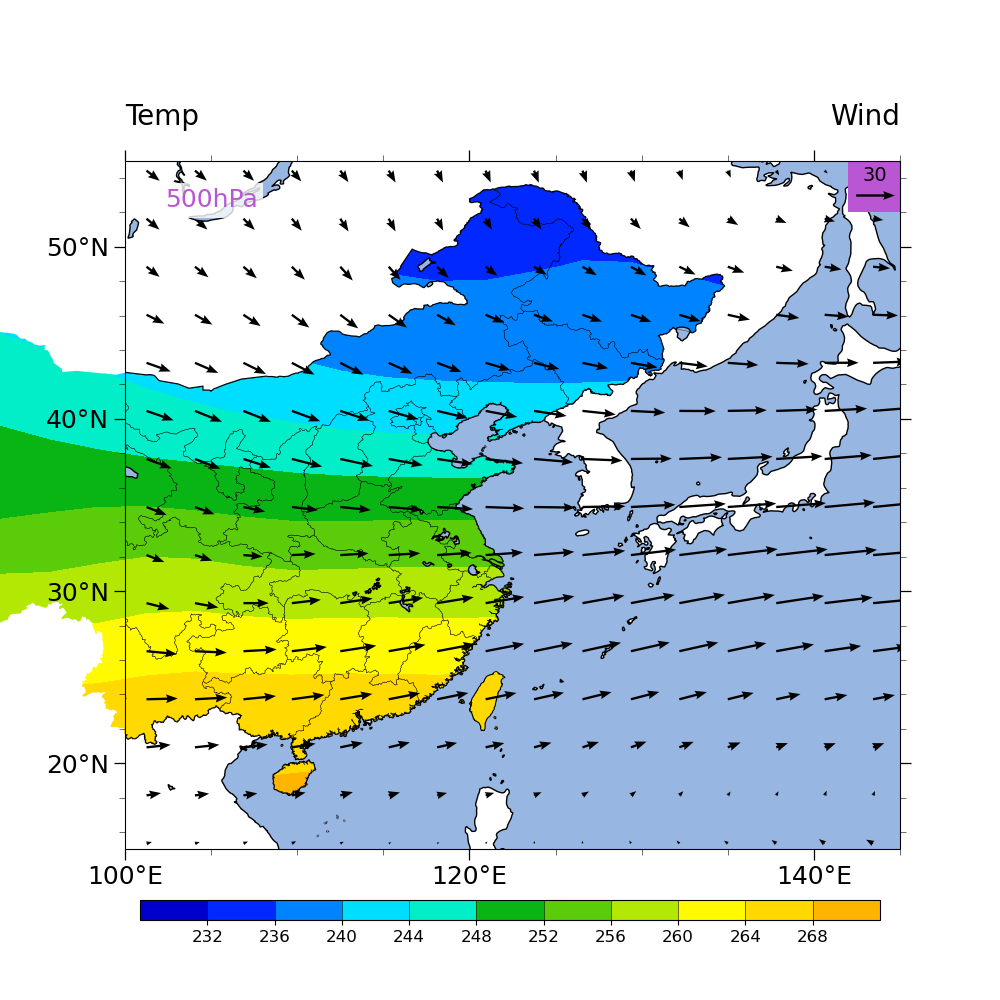# NCL_overlay_11b.py#

This script illustrates the following concepts:
• Overlaying vectors and filled contours on a map

• Masking out particular areas in a map

• Subsetting a color map

See following URLs to see the reproduced NCL plot & script:

This script shows how to overlay contours and vectors on a map, but with the contours limited to specific areas, and the vectors not limited.

The point of this script is to show how to mask contours against a geographical boundary, but in a way that allows them to be drawn up to the boundary location. This is unlike the shapefile masking examples, where grid points are set to missing if they fall outside a boundary, and hence you can get blocky features close to the boundary.

With Python’s matplotlib, there are 2 general approaches to accomplishing this:

1. You can “cover” (i.e., “over lay”) geographical features on top of other plots using the `zorder` parameter to most rendered objects.

2. You can “clip” a plot object with a geographical boundary.

This example demonstrates approach (b).

See NCL_overlay_11a.py for demonstration of approach (a).

Import packages:

```import xarray as xr
import numpy as np

from matplotlib import pyplot as plt
from matplotlib.patches import PathPatch

from cartopy.feature import ShapelyFeature, OCEAN, LAKES
from cartopy.crs import PlateCarree
from cartopy.mpl.patch import geos_to_path

import geocat.datafiles as gdf
import cmaps
import geocat.viz as gv
```

```# Open a netCDF data file using xarray default engine and load the data into xarrays, as well as extract slices for
# ``time=0`` and the ``lev=500`` hPa level
ds = xr.open_dataset(gdf.get("netcdf_files/uvt.nc")).sel(time=0, lev=500)

# For convenience only, extract the U,V,T and lat and lon variables
U = ds["U"]
V = ds["V"]
T = ds["T"]

lat = ds["lat"]
lon = ds["lon"]
```

## Construct shape boundaries#

Using Cartopy’s interface to the Natural Earth Collection of shapefiles and geographical shape data, we construct the geographical boundaries that we are interested in displaying, namely the country borders of China and Taiwan, the borders of Chinese provinces, and all land borders without China or Taiwan.

```# Download the Natural Earth shapefile for country boundaries at 10m resolution
shapefile = natural_earth(category='cultural',
resolution='10m',

# Sort the geometries in the shapefile into Chinese/Taiwanese or other
country_geos = []
other_land_geos = []
country_geos.append(record.geometry)
else:
other_land_geos.append(record.geometry)

# Define map projection to allow Cartopy to transform ``lat`` and ``lon`` values accurately into points on the
# matplotlib plot canvas.
projection = PlateCarree()

# Define a Cartopy Feature for the country borders and the land mask (i.e.,
# all other land) from the shapefile geometries, so they can be easily plotted
countries = ShapelyFeature(country_geos,
crs=projection,
facecolor='none',
edgecolor='black',
lw=1.5)
crs=projection,
facecolor='white',
edgecolor='none')

# Download the Natural Earth shapefile for the states/provinces at 10m resolution
shapefile = natural_earth(category='cultural',
resolution='10m',

# Extract the Chinese province borders
province_geos = [
record.geometry
]

# Define a Cartopy Feature for the province borders, so they can be easily plotted
provinces = ShapelyFeature(province_geos,
crs=projection,
facecolor='none',
edgecolor='black',
lw=0.25)
```

## Plot:#

```# Generate figure (set its size (width, height) in inches) and axes using Cartopy
fig = plt.figure(figsize=(10, 10))
ax = plt.axes(projection=projection)

ax.set_extent([100, 145, 15, 55], crs=projection)

# Draw the ocean and lake features

# Define the contour levels (T)
clevs = np.arange(228, 273, 4, dtype=float)

# Import an NCL colormap, truncating it by using geocat.viz.util convenience function
newcmp = gv.truncate_colormap(cmaps.BkBlAqGrYeOrReViWh200,
minval=0.1,
maxval=0.6,
n=len(clevs))

# Draw the contour plot, "clipped" to the country boundaries
# (NOTE: There are multiple closed polygons representing the boundaries of the
#        countries.  This is both because there are 2 country borders being used
#        to clip the contour plot, but also because China consists of many islands.
#        As a result, we have to loop over *all closed paths* and construct a
#        matplotlib patch object that we can use the clip the contour plot.)
for path in geos_to_path(country_geos):
patch = PathPatch(path,
transform=ax.transData,
facecolor='none',
edgecolor='black',
lw=1.5)

# Draw the patch on the plot

# Draw the contour plot
# (NOTE: Because this line is in the loop over closed paths, the contour plot
#        is being drawn for each closed path.  This has to be done because
#        matplotlib cannot apply *multiple* closed paths at the same time to
#        to the same plot.  Hence, for each closed path, we need to generate
#        another contour plot and clip that contour plot with the patch.  In
#        other words, every island on this plot corresponds to its own
#        contour plot!)
cf = ax.contourf(lon, lat, T, levels=clevs, cmap=newcmp)

# Clip each contour of the contour plot
# (NOTE: Each contour of the contour plot is actually its own "plot".  There
#        is no easy mechanism in matplotlib to clip the entire contour plot
#        at once, so we must loop through the "collections" in the contour
#        plot and clip each one separately.)
for col in cf.collections:
col.set_clip_path(patch)

cax = plt.axes((0.14, 0.08, 0.74, 0.02))
cbar = plt.colorbar(cf,
ax=ax,
cax=cax,
ticks=clevs[1:-1],
drawedges=True,
orientation='horizontal')
cbar.ax.tick_params(labelsize=12)

# Draw the province borders

# Draw the quiver plot (and its key)
Q = ax.quiver(lon,
lat,
U,
V,
color='black',
width=.003,
scale=600.,
rect = plt.Rectangle((142, 52),
3,
3,
facecolor='mediumorchid',
edgecolor=None,
zorder=1)
ax.quiverkey(Q,
0.9675,
0.95,
30,
'30',
labelpos='N',
color='black',
coordinates='axes',
fontproperties={'size': 14},
labelsep=0.1)

# Draw the '500hPa' label at the top left of the plot
props = dict(facecolor='white', edgecolor='none', alpha=0.8)
ax.text(105,
52.7,
'500hPa',
transform=projection,
fontsize=18,
ha='center',
va='center',
color='mediumorchid',
bbox=props)

# Use geocat.viz.util convenience function to set axes tick values
gv.set_axes_limits_and_ticks(ax,
xticks=[100, 120, 140],
yticks=[20, 30, 40, 50])

# Use geocat.viz.util convenience function to make plots look like NCL plots by using latitude, longitude tick labels

# Use geocat.viz.util convenience function to add minor and major tick lines
x_minor_per_major=4,
y_minor_per_major=5,
labelsize=18)

# Use geocat.viz.util convenience function to add main title as well as titles to left and right of the plot axes.
gv.set_titles_and_labels(ax,
lefttitle="Temp",
lefttitlefontsize=20,
righttitle="Wind",
righttitlefontsize=20)

# Show the plot
plt.show()
``````/home/docs/checkouts/readthedocs.org/user_builds/geocat-examples/conda/latest/lib/python3.10/site-packages/geocat/viz/util.py:692: UserWarning: Overwriting the cmap 'trunc(BkBlAqGrYeOrReViWh200,0.10,0.60)' that was already in the registry.
mpl.colormaps.register(new_cmap, force=force)
```

Total running time of the script: ( 0 minutes 14.312 seconds)

Gallery generated by Sphinx-Gallery